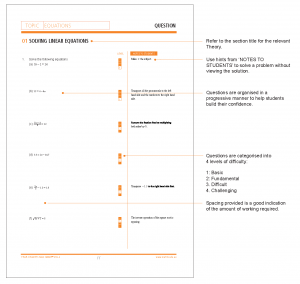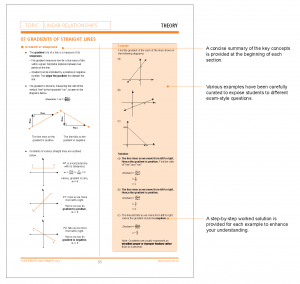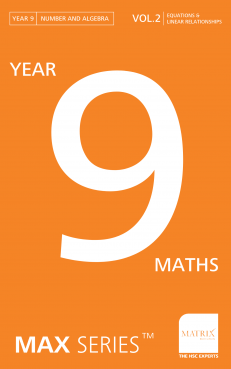# Year 9 Maths Max Series Vol 2: Equations & Linear Relationships Revision Workbook

Rated 5.00 out of 5 based on 1 customer rating
(1 customer review)

\$32.00

In stock

SKU: MTH9 Categories: ,

## This Year 9 Maths Revision Workbook for Equations and Linear Relationships is compliant with the NESA NSW Mathematics Stage 5.3 Syllabus.

The Maths revision content in the Year 9 Maths Max SeriesTM Volume 2 covers the topics ‘Equations and Linear Relationships’ from the ‘Number & Algebra’ strand of the NSW Mathematics Stage 5.2 and 5.3 Syllabus.

 Strand Topics Content Number and Algebra Equations Solving linear equations Rearranging formulas Rearranging equations Using equations to solve word problems Solving and graphing linear inequalities Linear Relationships Finding the midpoint and gradient of intervals Finding the distance between two points Graphing linear equations Finding the equation of a line parallel or perpendicular to a given line Solving simultaneous equations Using simultaneous equations to solve word problems

For detailed information including the contents of the topics, please visit the NESA website.

## The Year 9 Max SeriesTM  Volume 2 is not your ordinary Maths revision workbook.

Are you constantly surprised to find that your school exam questions differ from the textbook questions you spent hours perfecting? Do you find those practice papers your teacher provided for you didn’t quite prepare you for the exam?

Textbooks provide course content in a way that helps students gain a sound knowledge and understanding of the fundamental concepts.

But this is insufficient to achieve exam success at school as students are required to:

• Demonstrate extensive knowledge and skills
• Apply Maths concepts to unfamiliar questions/situations
• Use sophisticated multi-step reasoning
• Exhibit excellent problem-solving skills

## This Year 9 Maths revision workbook should be the last stop before your exam.

The Year 9 Maths Max SeriesTM is designed for maths revision and exam preparation, as well as providing students with the opportunity to further develop and practice the fundamental concepts. When it is used correctly, students will gain a significant advantage for their school exams.

The Year 9 Maths Max SeriesTM works by:

• Exposing students to exam-style questions to reduce the chance of encountering unfamiliar problems.
• Effectively preparing students for school exams by bridging the knowledge and skills gap between their textbooks and exam questions.
• Improving students’ problem-solving skills by sharing the techniques not published in textbooks.

## How to use this Year 9 Maths revision workbook.

Students should only use this maths revision workbook after gaining a sound knowledge and understanding of the key concepts of the Mathematics course content for equations and linear relationships. This book will effectively prepare students for school exams when the following steps are closely followed.

Step 1 Review theory content

At the beginning of each topic, a concise summary of the key concepts is provided. Be sure to read and understand it before attempting any questions.Step 2 Study the examples and their solutions to gain clarity

The examples expose students to various exam-style questions. The step-by-step worked solutions for each example provides clear multi-step reasoning to enhance your understanding and provide a structure for maximising marks in exams.

Step 3 Attempt practice questions to improve problem-solving skills

Questions are organised in a progressive manner to facilitate independent learning.

If you have difficulty, the ‘NOTES TO STUDENTS’ section provides you with hints to encourage problem solving without referring to the solution.

The ‘LEVEL’ of difficulty is a useful tool to assess your ability to solve new, unseen and difficult problems.## 1 review for Year 9 Maths Max Series Vol 2: Equations & Linear Relationships Revision Workbook

1.Rated 5 out of 5

Jane Lim

My daughter found this book was great for exam preparation! Love the hints section so she can self help with difficult questions.You're viewing: Year 9 Maths Max Series Vol 2: Equations & Linear Relationships Revision Workbook \$32.00
Rated 5.00 out of 5